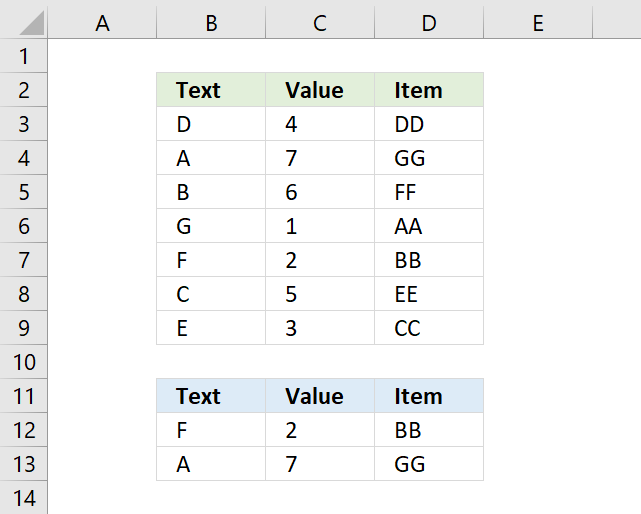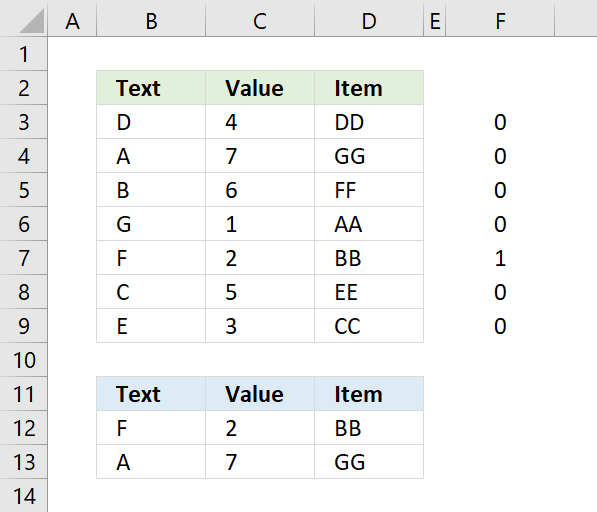Author: Oscar Cronquist Article last updated on August 21, 2018

###The array formula in cell D12 matches two values in two columns each and returns a value on the same row.

If you are looking for a way to compare two columns for differences or compare two columns for same values, please click links.

You are not limited to formulas, conditional formatting allows you to compare two columns and highlight matches or compare two columns and highlight differences.

=INDEX(\$D\$3:\$D\$9, MATCH(1, COUNTIFS(B12, \$B\$3:\$B\$9, C12,\$C\$3:\$C\$9) ,0))

To enter an array formula, type the formula in a cell then press and hold CTRL + SHIFT simultaneously, now press Enter once. Release all keys.

The formula bar now shows the formula enclosed with curly brackets telling you that you entered the formula successfully. Don't enter the curly brackets yourself.

The COUNTIFS function counts rows where both values match, however, it returns an array that corresponds to the number of rows in cell range B3:D9.

COUNTIFS(B12, \$B\$3:\$B\$9, C12,\$C\$3:\$C\$9)

returns {0;0;0;0;1;0;0} , shown in column F in picture below.Both values match in row 7.

MATCH(1, COUNTIFS(B12, \$B\$3:\$B\$9, C12,\$C\$3:\$C\$9) ,0)

The MATCH function identifies the relative position of the matching values.

MATCH(1, {0;0;0;0;1;0;0} ,0)

and returns 5, the match is the fifth value in the array.

INDEX(\$D\$3:\$D\$9, MATCH(1, COUNTIFS(B12, \$B\$3:\$B\$9, C12,\$C\$3:\$C\$9) ,0))

The INDEX function returns the corresponding value in column D.

INDEX(\$D\$3:\$D\$9, 5)

and returns BB in cell D12.

### Alternative regular formula

=INDEX(\$D\$3:\$D\$9, MATCH(1, INDEX(COUNTIFS(B12, \$B\$3:\$B\$9, C12, \$C\$3:\$C\$9), ), 0))

The above formula is a regular formula, it is slightly larger than the first formula at the beginning of this post.

Match two columns.xlsx

### Compare two columns for differences

This article extracts values that only exists in one column:

Compare two columns and return differences

The image above demonstrates an array formula in cell B11 that extracts values that only exist in List 1 (B3:B7) […]

### Compare two columns for same values

Extract shared values between two columns

Question: How can I compare two columns to find values that exists in both cell ranges? The picture above shows […]

### Compare two columns and highlight matches

Compare two columns and highlight values in common

A conditional formatting formula highlights values in column B that also exist in column D. =COUNTIF(\$D\$3:\$D\$7,B3) The same thing happens […]

### Match two columns and return a third

The following article is related and demonstrates how to find two values that match on the same row and return a third value on the same row:

INDEX MATCH with multiple criteria

The formula demonstrated in cell D13 is a regular formula, most people prefer a regular formula over an array formula […]